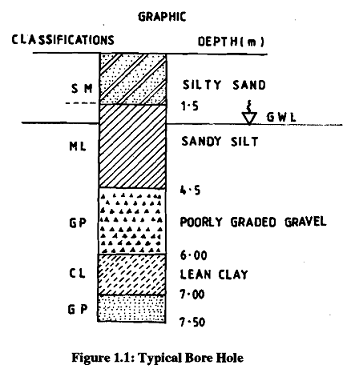## State the location and spacing of borings, Civil Engineering

Assignment Help:

Location and spacing of borings

The location, spacing of geophysical profiles, soundings and borings should be such that,  you will be in a position to accurately predict the characteristics of underlying stratum. You must also be in a position to disclose the important variations in the sub-surface condition#### Steel design, what is tension member

what is tension member

#### Load transmission, • The transmission of load through a structure can be co...

• The transmission of load through a structure can be complex but in any particular case            - The applied load can usually be classified into 4 types                •

#### Arches, construction of arches

construction of arches

#### What is the correct value of the specific gravity, In a specific gravity te...

In a specific gravity test, 1.17 N of oven-dired soil was taken. The weight of pyknometer, soil and water was obtained as 6.51 N. The weight of pyknometer full of water alone was 5

#### Explain total dynamic head (tdh), Total Dynamic Head: (TDH) y-axis plo...

Total Dynamic Head: (TDH) y-axis plot point related to each x-axis flow rate along the system curve plot. TDH = h a = TSH + h f + v 2 /2g       v 2 / 2g usually small

#### Explain the equation for length of spiral curve, Explain the Equation for L...

Explain the Equation for Length of Spiral Curve Length of Spiral Curve can be calculated using the following equation L s = 3.15V 3 /RC Where: V - Design Speed in MPH

#### EDTA, how to find total hardness by EDTA

how to find total hardness by EDTA

#chain

#### Design, what is the procedure to design the beam and column?

what is the procedure to design the beam and column?

#### Determine the steps for routine inspection, Determine the Steps for routine...

Determine the Steps for routine inspection? A routine inspection should normally include: - A Level I inspection on 100% of the underwater portion of the structure to determ